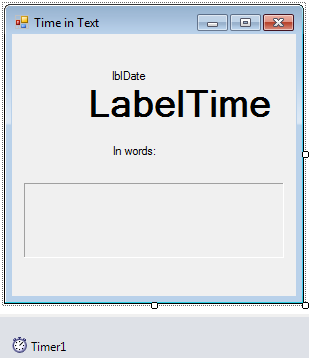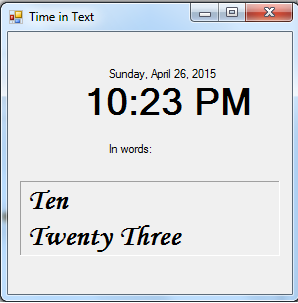# Convert Time into Text in VB.NET

Today, I will teach you how to convert time into text using VB.NET because usually we always display the time in numbers.

Now, let's start this tutorial!

1. Let's start with creating a Windows Form Application for this tutorial by following the following steps in Microsoft Visual Studio: Go to File, click New Project, and choose Windows Application.

2. Next, add labels named lblDate for displaying the date, LabelTime for displaying the time in numbers and LabelTimeInText for displaying the time in text. Insert also a Timer named Timer1 for accessing the Time. You must design your interface like this:3. Then we will code for our Form.

We will have first a function named TimeInText(ByVal CurrentHour As Int32, ByVal CurrentMinute As Int32) As String to display the hour of the current time.

`   Function TimeInText(ByVal CurrentHour As Int32, ByVal CurrentMinute As Int32) As String        Dim Hour, Min As Int32        Dim ClockInText As String        Hour = CurrentHour        Min = CurrentMinute        If Hour = 1 Or Hour = 13 Then            ClockInText = "One"        ElseIf Hour = 2 Or Hour = 14 Then            ClockInText = "Two"        ElseIf Hour = 3 Or Hour = 15 Then            ClockInText = "Three"        ElseIf Hour = 4 Or Hour = 16 Then            ClockInText = "Four"        ElseIf Hour = 5 Or Hour = 17 Then            ClockInText = "Five"        ElseIf Hour = 6 Or Hour = 18 Then            ClockInText = "Six"        ElseIf Hour = 7 Or Hour = 19 Then            ClockInText = "Seven"        ElseIf Hour = 8 Or Hour = 20 Then            ClockInText = "Eight"        ElseIf Hour = 9 Or Hour = 21 Then            ClockInText = "Nine"        ElseIf Hour = 10 Or Hour = 22 Then            ClockInText = "Ten"        ElseIf Hour = 11 Or Hour = 23 Then            ClockInText = "Eleven"        ElseIf Hour = 0 Or Hour = 12 Then            ClockInText = "Twelve"        End If        If Min < 10 Then            ClockInText &= vbNewLine & "    O' " & MinInText(Min)        Else            ClockInText &= " " & vbNewLine & MinInText(Min)        End If        Return ClockInText    End Function`

Then for the minute to display, we will create another function named
MinInText (ByVal Min As Int32) As String

`    Function MinInText(ByVal Min As Int32) As String        Dim MiT As String, cMin As Int32        cMin = Min        If cMin = 1 Then            MiT = "One"        ElseIf cMin = 2 Then            MiT = "Two"        ElseIf cMin = 3 Then            MiT = "Three"        ElseIf cMin = 4 Then            MiT = "Four"        ElseIf cMin = 5 Then            MiT = "Five"        ElseIf cMin = 6 Then            MiT = "Six"        ElseIf cMin = 7 Then            MiT = "Seven"        ElseIf cMin = 8 Then            MiT = "Eight"        ElseIf cMin = 9 Then            MiT = "Nine"        ElseIf cMin = 10 Then            MiT = "Ten"        ElseIf cMin = 11 Then            MiT = "Eleven"        ElseIf cMin = 12 Then            MiT = "Twelve"        ElseIf cMin = 13 Then            MiT = "Thirteen"        ElseIf cMin = 14 Then            MiT = "Fourteen"        ElseIf cMin = 15 Then            MiT = "Fifteen"        ElseIf cMin = 16 Then            MiT = "Sixteen"        ElseIf cMin = 17 Then            MiT = "Seventeen"        ElseIf cMin = 18 Then            MiT = "Eighteen"        ElseIf cMin = 19 Then            MiT = "Nineteen"        ElseIf cMin = 20 Then            MiT = "Twenty"        ElseIf cMin = 21 Then            MiT = "Twenty One"        ElseIf cMin = 22 Then            MiT = "Twenty Two"        ElseIf cMin = 23 Then            MiT = "Twenty Three"        ElseIf cMin = 24 Then            MiT = "Twenty Four"        ElseIf cMin = 25 Then            MiT = "Twenty Five"        ElseIf cMin = 26 Then            MiT = "Twenty Six"        ElseIf cMin = 27 Then            MiT = "Twenty Seven"        ElseIf cMin = 28 Then            MiT = "Twenty Eight"        ElseIf cMin = 29 Then            MiT = "Twenty Nine"        ElseIf cMin = 30 Then            MiT = "Thirty"        ElseIf cMin = 31 Then            MiT = "Thirty One"        ElseIf cMin = 32 Then            MiT = "Thirty Two"        ElseIf cMin = 33 Then            MiT = "Thirty Three"        ElseIf cMin = 34 Then            MiT = "Thirty Four"        ElseIf cMin = 35 Then            MiT = "Thirty Five"        ElseIf cMin = 36 Then            MiT = "Thirty Six"        ElseIf cMin = 37 Then            MiT = "Thirty Seven"        ElseIf cMin = 38 Then            MiT = "Thirty Eight"        ElseIf cMin = 39 Then            MiT = "Thirty Nine"        ElseIf cMin = 40 Then            MiT = "Forty"        ElseIf cMin = 41 Then            MiT = "Forty One"        ElseIf cMin = 42 Then            MiT = "Forty Two"        ElseIf cMin = 43 Then            MiT = "Forty Three"        ElseIf cMin = 44 Then            MiT = "Forty Four"        ElseIf cMin = 45 Then            MiT = "Forty Five"        ElseIf cMin = 46 Then            MiT = "Forty Six"        ElseIf cMin = 47 Then            MiT = "Forty Seven"        ElseIf cMin = 48 Then            MiT = "Forty Eight"        ElseIf cMin = 49 Then            MiT = "Forty Nine"        ElseIf cMin = 50 Then            MiT = "Fifty"        ElseIf cMin = 51 Then            MiT = "Fifty One"        ElseIf cMin = 52 Then            MiT = "Fifty Two"        ElseIf cMin = 53 Then            MiT = "Fifty Three"        ElseIf cMin = 54 Then            MiT = "Fifty Four"        ElseIf cMin = 55 Then            MiT = "Fifty Five"        ElseIf cMin = 56 Then            MiT = "Fifty Six"        ElseIf cMin = 57 Then            MiT = "Fifty Seven"        ElseIf cMin = 58 Then            MiT = "Fifty Eight"        ElseIf cMin = 59 Then            MiT = "Fifty Nine"        Else            MiT = " Clock"        End If        Return MiT    End Function`

We will code for the Timer1_Tick for displaying the Date, Time in Numbers and Time in Text using the functions that we declared above.

`    Private Sub Timer1_Tick(ByVal sender As System.Object, ByVal e As System.EventArgs) Handles Timer1.Tick        LabelTime.Text = Now.ToShortTimeString        LabelDate.Text = Now.ToLongDateString        LabelTimeInText.Text = TimeInText(Now.Hour, Now.Minute)    End Sub`

Then enable the time in the Form_Load.

`    Private Sub Form1_Load(ByVal sender As System.Object, ByVal e As System.EventArgs) Handles MyBase.Load        Timer1.Enabled = True    End Sub`

### Output:For more inquiries and need programmer for your thesis systems in any kind of programming languages, just contact my number below.

Best Regards,

Engr. Lyndon R. Bermoy
IT Instructor/System Developer/Android Developer
Mobile: 09079373999
Telephone: 826-9296
E-mail:[email protected]

Subscribe at my YouTube Channel at: Ser Bermz

Visit my website at: www.campcodes.com

### Tags

Note: Due to the size or complexity of this submission, the author has submitted it as a .zip file to shorten your download time. After downloading it, you will need a program like Winzip to decompress it.

Virus note: All files are scanned once-a-day by SourceCodester.com for viruses, but new viruses come out every day, so no prevention program can catch 100% of them.